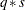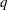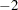The CATMOD Procedure
 Displayed Output

PROC CATMOD displays the following information in the "Data Summary" table:

• the response effect

• the weight variable, if one is specified

• the data set name

• the number of response levels

• the number of samples or populations

• the total frequency, which is the total sample size

• the number of observations from the data set (the number of data records)

• the frequency of missing observations, labeled as "Frequency Missing"

Except for the analysis of variance table, all of the following items can be displayed or suppressed, depending on your specification of statements and options.

• The ONEWAY option produces the "One-Way Frequencies" table, which displays the frequencies of each variable value used in the analysis.

• The populations (or samples) are defined in a table labeled "Population Profiles." The sample size and the values of the defining variables are displayed for each sample. This table is suppressed if the NOPROFILE option is specified.

• The observed responses are defined in a table labeled "Response Profiles." The values of the defining variables are displayed for each response. This table is suppressed if the NOPROFILE option is specified.

• If the FREQ option is specified, then the "Response Frequencies" table is displayed, which shows the frequency of each response for each population.

• If the PROB option is specified, then the "Response Probabilities" table is produced. This table displays the probability of each response for each population.

• If the COV option is specified, the "Response Functions, Covariance Matrix" table, which shows the covariance matrix of the response functions for each sample, is displayed.

• If the DESIGN option is specified, the response functions are displayed in the "Response Functions, Design Matrix" table. If the COV option is also specified, the response functions are displayed in the "Response Functions, Covariance Matrix" table.

• If the DESIGN option is specified, the design matrix is displayed in the "Response Functions, Design Matrix" table, and if a log-linear model is being fit, the _RESPONSE_ matrix is displayed in the "_Response_ Matrix" table. If the model type is AVERAGED, then the design matrix is displayed withrows, assumingresponse functions for each ofpopulations. Otherwise, the design matrix is displayed with onlyrows since the model is the same for each of theresponse functions.

• The "X*Inv(S)*X" matrix is displayed for weighted least squares analyses if the XPX option is specified.

• The "Analysis of Variance" table for the weighted least squares analysis reports the results of significance tests for each of the design-effects on the right side of the MODEL statement. If _RESPONSE_ is a design-effect and is defined explicitly in the LOGLIN, FACTORS, or REPEATED statement, then the table contains test statistics for the individual effects constituting the _RESPONSE_ effect. If the design matrix is input directly, then the content of the displayed output depends on whether you specify any subsets of the parameters to be tested. If you specify one or more subsets, then the table contains one test for each subset. Otherwise, the table contains one test for the effect MODEL | MEAN. In every case, the table also contains the residual goodness-of-fit test. Produced for each test of significance are the source of variation, the number of degrees of freedom (DF), the Wald chi-square value, and the significance probability (Pr > ChiSq).

• The "Analysis of Weighted Least Squares Estimates" table lists, for each parameter in the model, the least squares estimate, the estimated standard error of the parameter estimate, the Wald chi-square value (calculated as ((parameter estimate)/(standard error))) for testing that the parameter is zero, and the significance probability (Pr > ChiSq) of the test. If the CLPARM option is specified, then 95% Wald confidence intervals are displayed.

Each row in the table is labeled with the parameter (the model effect and the class levels) and the response function number; however, if the NOPREDVAR option or a REPEATED or FACTORS statement is specified or if the design matrix is directly input, the rows are labeled by the effect in the model for which parameters are formed and the parameter number.

• The "Covariance Matrix of the Parameter Estimates" table for the weighted least squares analysis displays the estimated covariance matrix of the least squares estimates of the parameters, provided that the COVB option is specified.

• The "Correlation Matrix of the Parameter Estimates" table for the weighted least squares analysis displays the estimated correlation matrix of the least squares estimates of the parameters, provided that the CORRB option is specified.

• The "Maximum Likelihood Analysis" table is produced when the ML and ITPRINT options are specified for the standard response functions. It displays the iteration number, the number of step-halving sub-iterations,log likelihood for that iteration, the convergence criterion, and the parameter estimates for each iteration.

• The "Maximum Likelihood Analysis of Variance" table, displayed when the ML option is specified for the standard response functions, is similar to the table produced for the least squares analysis. The Wald chi-square test for each effect is based on the information matrix from the likelihood calculations. The likelihood ratio statistic compares the specified model with the unrestricted (saturated) model and is an appropriate goodness-of-fit test for the model.

• The "Analysis of Maximum Likelihood Estimates" table, displayed when the ML option is specified for the standard response functions, is similar to the one produced for the least squares analysis. The table includes the maximum likelihood estimates, the estimated standard errors based on the information matrix, and the Wald chi-square statistics based on estimated standard errors.

• The "Covariance Matrix of the Maximum Likelihood Estimates" table displays the estimated covariance matrix of the maximum likelihood estimates of the parameters, provided that the COVB and ML options are specified for the standard response functions.

• The "Correlation Matrix of the Maximum Likelihood Estimates" table displays the estimated correlation matrix of the maximum likelihood estimates of the parameters, provided that the CORRB and ML options are specified for the standard response functions.

• For each source of variation specified in a CONTRAST statement, the "Contrasts" table lists the label for the source (Contrast), the number of degrees of freedom (DF), the Wald chi-square value, and the significance probability (Pr > ChiSq). If the ESTIMATE= option is specified, the "Analysis of Contrasts" table displays, for each row of the contrast, the label (Contrast), the type (PARM or EXP), the row of the contrast, the estimate and its standard error, a Wald confidence interval, the Wald chi-square, and the p-value (Pr > ChiSq) for 1 degree of freedom.

• Specification of the PREDICT option in the MODEL statement has the following effect. Produced for each response function within each population are the observed and predicted function values, their standard errors, and the residual (observed minus predicted). If the response functions are the default ones (generalized logits), additional information displayed for each response within each population includes the observed and predicted cell probabilities, their standard errors, and the residual. However, specifying PRED=FREQ in the MODEL statement results in the display of the predicted cell frequencies rather than the predicted cell probabilities. The displayed output includes the population profiles and, for the response function table, the function number, while the probability and frequency tables display the response profiles. If the NOPREDVAR option is specified in the MODEL statement, the population profiles are replaced with the sample numbers, and the response profiles are replaced with the labels "P" for theth cell probability, and "F" for theth cell frequency.

• When there are multiple RESPONSE statements, the output for each statement starts on a new page. For each RESPONSE statement, the corresponding title, if specified, is displayed at the top of each page.

• If the ADDCELL= option is specified in the MODEL statement, and if there is a weighted least squares analysis specified, the adjusted sample size for each population (with number added to each cell) is labeled "Adjusted Sample Size" in the "Population Profiles" table. Similarly, the adjusted response frequencies and probabilities are displayed in the "Adjusted Response Frequencies" and "Adjusted Response Probabilities" tables, respectively.

• If _RESPONSE_ is defined explicitly in the LOGLIN, FACTORS, or REPEATED statement, then the definition is displayed as a note whenever _RESPONSE_ appears in the output.Previous Page | Next Page | Top of Page